## Help with polynomial zeros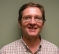Started by 2 years ago27 replieslatest reply 2 years ago193 views

I'm working on a problem where I need a *very* long FIR with many stop bands.  I've done several similar designs recently and remez() with the right prodding will give the desired response.  This one is just too big and after hours of crunching it wont converge.

So OK, back to basic principles.  I can put a pair of zeros on the unit circle by computing their location on the complex plane with RootsOfZeros = cos(f*w) +/- i*sin(f*w); where w = omega.  Taking the output of a small FIR from remez() I can go back and forth from roots to the impulse response with roots() and poly() and the locations are fixed.  There is no problem from rounding errors.  I can look at the locations with zplane();

So I can place multiple zero pairs on the unit circle by multiplying the small polynomials together and check the impulse response with zplane() and freqz().  This works until about the 4th one.  Then I start getting wildly different answers.  The zeros have moved around on the complex plane.  That means the roots of the output polynomial are different than the roots of the inputs.  Sure enough roots() gives me different roots than what I started with.  Not just scaling, they are in different places.

At a loss here.  Obviously there is some basic bit of math that I am missing.

The goal is to synthesize a polynomial in Z (FIR impulse response) with zero pairs I pick on the plane.

Below is a bit of Matlab code I was tinkering with.

If I do 4 zeros then zooming in on the z plane shows the zeros nicely placed where they should go.  Add the fifth zero pair and they split.  Add the sixth and all the zeros are arranged in a rosette about +1 on the complex plane.  Any ideas?

Fb = 4.2e6;

Fs = 4.321e9;

Fs_dec = Fs/125;

Fst_1a = Fs_dec - Fb;   % Lower Edge of 1st pass band image

Fst_1b = Fs_dec + Fb;   % Upper Edge of 1st pass band image

Fst_2a = 2*Fs_dec - Fb; % Lower Edge of 2nd pass band image

Fst_2b = 2*Fs_dec + Fb; % Upper Edge of 2nd pass band image

frb(1) = Fst_1a;

frb(2) = Fs_dec;

frb(3) = Fst_1b;

frb(4) = Fst_2a;

frb(5) = 2*Fs_dec;

frb(6) = Fst_2b;

b = [1 1]; % Zero at Z = -1

% for n = 1:6

% for n = 1:4

for n = 3:6

rbp = cos(frb(n)*2*pi/Fs) + i*sin(frb(n)*2*pi/Fs);

rbm = cos(frb(n)*2*pi/Fs) - i*sin(frb(n)*2*pi/Fs);

pol = poly([rbp rbm]);

b = conv(b, pol);

b = b/sum(b);

end

figure(1);freqz(b/sum(b),1,2^21,Fs);

figure(2);zplane(b,1);grid;

[ - ]I'm enjoying this because it's making me think of something I haven't considered before.

As a polynomial's order increases, the locations of the roots become ever more sensitive to variations in the parameters.  This fact -- or one of its many corollaries -- is the reason that when you're implementing IIR filters you always cascade 1st- or 2nd-order sections, and why you don't let the sampling rate get too high.

The light bulb that just went off for me is that this works for FIR filter zeros, too!  D'oh!  So if you're looking for zeros in the response that are as close as possible to zero, you can't let the polynomial order get too high.

So, it may be that if the number and depth of the zeros are important, you may need to recognize that you're facing a fundamental limitation in your problem, and you may need to cascade a number of FIR filters.  Depending on just how sensitive your system is to signal content in those notches, you may have to pay very close attention to numerical precision while you're at it.

[ - ]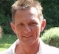A long time ago I had to tune a fiddly multistage radio filter (HW).
I was very successful with a single stage filter, and even with two-stage filters. However with more stages I ended up with always correcting the former stages over and over again - and finally just dropped my goal :)

Later I realized that my problems where quite common - it IS difficult.
And even if I do a good job, the result depends on so many details (like temperature effects).

But my analogue experiences helped me to understand that not only the concept of filtering is transferred to the digital realm - but the sensitivity and trickiness follow.

Always.

Bernhard

[ - ]Hello Napierm,

Here is the problem: the factored form of the polynomial P(x) = product(x-root(k)) k=1,2,...., N  and the expanded form p(x) = sum a(n)x^(N-n), n=0, 1, 2, .... N, with a(0)=1, a(1)=sum of the roots, a(2)=sum of roots taken 2 at a time,... and a(N)=product of roots,  can not be the same in a finite state machine.

First problem is that roots on the unit circle are transcendental numbers and require infinite length words.  If the roots can be represented by finite length words each successive product in forming the filter coefficients get longer and longer and for sufficiently high order polynomials you are guaranteed to run out of bits in your coefficient word length. When the coefficients are replaced by their finite length approximations the roots move and the roots you finally get are not the roots with which you started.

There are ways of approximating your spectrum with well behaved filter coefficients that require some thinking outside the box.

Why not pass on our filter specs and let me have a look see at one of my approaches.

fred harris

fjharris@ucsd.edu

[ - ]Remez, firls ...etc are based on iterations to converge and so I expect they have limit on number of taps to handle.

Using fir2(frequency grid method, internally does ifft) I just used 1000 taps here:

h = fir2(1000,[0 .1 .15 .2 .23 .25 .26 .3 .33 .35 .4 1],[1 1 0 0 1 1 0 0 1 1 0 0]);

zplane(h,1)

hquant = round(2^18 * h);

you can go higher than 1000... at the edges the coeffs get really tiny and need wider bitwidth if using fixed point.

[ - ]I'm not very familiar with Matlab.  In some coding I've been doing I found "long double" is a 128 bit float.  Can you tell Matlab to use more bits?  Your description sounds like Nth roots of 1 which would be exp(2*Pi*j/N) for j integer.  If the numbers are getting very close to each other, it could be a round off issue.

Instead of unit circle, scale the roots to be around 1/2^21.  Maybe that will keep the numbers in a range the algorithm can deal with.  I have no idea if that will do anything useful!

Mike

[ - ]Yes, I'm considering round off.  Just doesn't seem like that is the problem and Matlab doesn't natively support any float bigger than a double. This is not trivial to work around.

Just to try something I'm looking at the HPF (High Precision Floating point) library.  It does support arbitrarily high precision numbers but is pretty basic.  No complex types for instance.  Fortunately there are only a few forms for the complex conjugate pairs in the pass band and stop band that all reduce to simple real coefficients.  Then the polynomial multiplication is done by convolution.

The examples I've worked by hand agree with both poly() and conv().  So I can write a routine to do this.  Just tedious to see if round off is the problem or not.

[ - ]I've worked a few more examples with HPF and seems like my intuition is correct.  The problem is not lack of precision.  Increasing to a crazy number of decimal digits (more than 1000) doesn't alter the outcome at all.  Not even a marginal difference.  There is something going on here I'll have to dig in to understand.  It goes counter to my experience.  Normally you can take two responses and use convolution combine them.

BTW the example in the Matlab snippet is a simpler one that remez() already successfully solves.  It has *no* trouble concentrating zeros much more tightly packed and in the same locations on the unit circle as in this Matlab code.

Have to admit I can only infer that from the frequency response looking at the attenuation pits.  zplane() shows garbage - it can't factor the output correctly.

The time hasn't been a total waste.  I've worked out cases of how to place zeros and how they convert to simple polynomials.  Turns out you can take a standard window function and alter it with convolution to tweek it a bit.  I can get to a solution this way but is much lower performance than remez().  I have a couple of outputs that would work but  are "suboptimal"; means takes more coefficients than I want to store in memory.

Side note: one issue is that the polynomial solution to the zero pair placements results in only cos(angle) being used.  In all cases i*sin(angle) terms cancel out.  That means that the angle resolution near DC gets very sensitive.

My next notion going down this rabbit hole is to dig into the papers on the P-M algorithm of how they move the extremal frequencies around to approximate a response.

[ - ]Have you tried FIR design methods like DRHS-OLL?

[ - ]The results do look interesting.  I don't see that the code base has been made available for others to try.

[ - ]That's great!  It's a major pain of course because you're not getting the simple answer quickly, but understanding how all the programs actually work and why they go bonkers will pay off for a long time.  Matlab drives me nuts, so I don't use it unless I have no choice.  But it is a good tool.  Good luck going down the rabbit hole - at least you will have fun along the way!

[ - ]Years ago, I ran into a problem because Matlab's root() algorithm was not able to give proper solutions just because of the internal math precision.

Meanwhile I abandoned Matlab because of that, but at that time (some 15 years ago), Matlab insiders explained me that the math precision mainly depends on the machine on which it's running: on a 64bit machine it's better than on a 32bit machine. Because it relies on the underlying hardware - (a sacrifice to speed on customers' demand).

My guess is that the problem here is still due to insufficient precision within some basic math functions like root(). Therefore all dependent algorithms in Matlab which rely on those basic algorithms (like check for stability if roots/zeros are very close to the unit circle) may suffer.

Switching to ScopeFIR instead of Matlab got me much more reliable results.
I don't know if you can/want to give it a try.
Otherwise it might help to write your own algorithm which is able to
work with arbitrary precision (like Fortran, Numpy, ...) and then try with
increasing precision. Important is that the whole algorithm is working with this increased precision. Matlab is definitely not the right tool for this.
(Except if it has a toolbox which supports this, meanwhile ...)

Bernhard

[ - ]ScopeFIR looks interesting.  But right off is says the limitation for number of taps is 16K.  Not big enough.  Another is that I almost always wind up with a script with a bunch of constants at the top, knobs I call them.  I turn the knobs to tweak the response.  Or where some parameter is really sensitive I have a loop that can grind away looking for a minimum.  Or do a gradient descent.  That is hard with any canned tool that doesn't allow me to script it and get "under the hood".

Here's one example from a past job.  There are two cascaded CIC's that are modified for better attenuation of a problem frequency.  The output passband shape is not flat or a standard CIC droop.  I can compensate with a modified half-band at the last decimation stage.  It's easy to do with remez().  Make many closely spaced but not touching passbands in your actual passband.  Set each one to the gain needed to compensate.  Note the beginning and ending gains of each sub-band can be different so I just set them to what I want at each frequency.  This does dork about with the stop-band attenuation shape due to half-band symmetry but if it attenuates enough then who cares.

[ - ]If you like ScopeFIR, you might want to contact the developer.
Few years ago he was very communicative - so why not ask him for a specialized version with higher limits...

[ - ]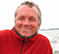Hi,

your 'for' loop gets much shorter with these two lines

ff1 = (exp(j*2*pi*[frb -frb]/Fs));
fff = poly(ff1);

But that does not bring you out of the numeric troubles with filter design. The abs(roots(fff)) range from 0.98 to 1.03. You will also get in trouble with the precision and accuracy for the resulting FIR.

The zeros are too close together. You bring them apart by reducing the decimation :

Fs_dec = Fs/25;

Cheers

Detlef

[ - ]Yes, that does make all 6 zero pairs plot correctly.  Doesn't solve the problem that I need a metric crap ton of them close together on the circle.  But does point to maybe round off error as the problem.  So will keep poking at this HPF code.

Thanks,

Mark

[ - ]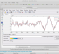Trick for ff1 without loop solution is nice.

Yes, the roots are too close, stopband bandwidth (2xFb/Fs ~ 0.002) is also too low compared to sampling rate.

[ - ]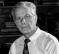Hello

try to use the function poly:

>> help poly

poly Convert roots to polynomial.

poly(A), when A is an N by N matrix, is a row vector with

N+1 elements which are the coefficients of the

characteristic polynomial, det(lambda*eye(size(A)) - A).

poly(V), when V is a vector, is a vector whose elements are

the coefficients of the polynomial whose roots are the

elements of V. For vectors, ROOTS and poly are inverse

functions of each other, up to ordering, scaling, and

roundoff error.

regards

[ - ]Respectfully, I've tried poly and conv.  They yield identical results.

[ - ]Hi,

even after b/sum(b), coefficients of b remain high;

1.0e+15 *

Columns 1 through 8

0.0183   -0.2008    0.9831   -2.7972    4.9864   -5.3791    2.3893    2.3893

Columns 9 through 14

-5.3791    4.9864   -2.7972    0.9831   -0.2008    0.0183

[ - ]Nutty, yes?

[ - ]Actually we should normalize 'b' by b in the loop (and after the loop, not by sum(b)), then we get the same result suggested without for loop;

without for  (added zero for Fs/2)
ff1 = (exp(j*2*pi*[frb -frb Fs/2]/Fs));
fff = real(poly(ff1))

we get ;
Columns 1 through 8
1.0000  -10.9620   53.6584 -152.6732  272.1596 -293.5937  130.4109  130.4109
Columns 9 through 14
-293.5937  272.1596 -152.6732   53.6584  -10.9620    1.0000



for the other case;

If we normalize 'b' by b in the loop and also after the loop (instead of sum(b));

b =
Columns 1 through 8
1.0000  -10.9620   53.6584 -152.6732  272.1596 -293.5937  130.4109  130.4109

Columns 9 through 14
-293.5937  272.1596 -152.6732   53.6584  -10.9620    1.0000

[ - ]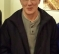Napierm-

Possibly foolish answer here, but since you need stopbands, what about 2 filters in series ?  Maybe that does something to delay for particular stopbands that is not good for your application, but at least you might get Matlab's Remez algorithm to converge.

-Jeff

[ - ]Not foolish at all.  Unfortunately the form of this particular problem hits a large prime number so the exact solution needs the long FIR.  Yes, there are other ways to skin the cat but would require *much* more hardware.  I can't really give more information.

[ - ]Napierm-

Ok ... sounds like a maximum length sequence problem or encryption related. Can you find where in the MATLAB source it fails to converge, and start instrumenting that ?

-Jeff

[ - ]I don't have the source to Matlab's remez() or firpm() code.  Regardless it is still likely to cough up on the ~20K point FIR.  Foolish of me to try.

Thanks,

Mark

[ - ]Mark-

Huh ... I thought MATLAB had open sourced everything. I guess they still subscribe to the Steve Ballmer school of thought about Linux being communism -- they are from the same era, haha.

-Jeff

[ - ]